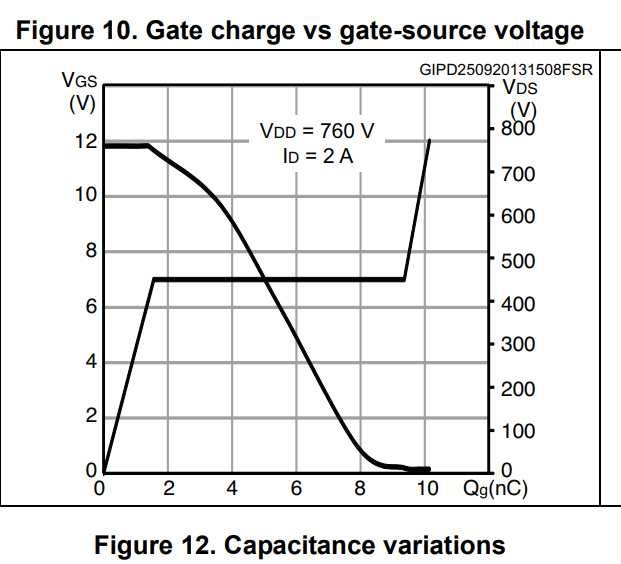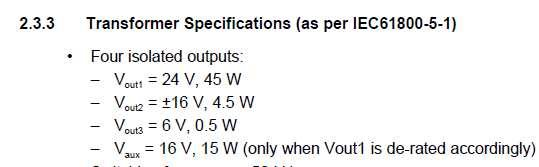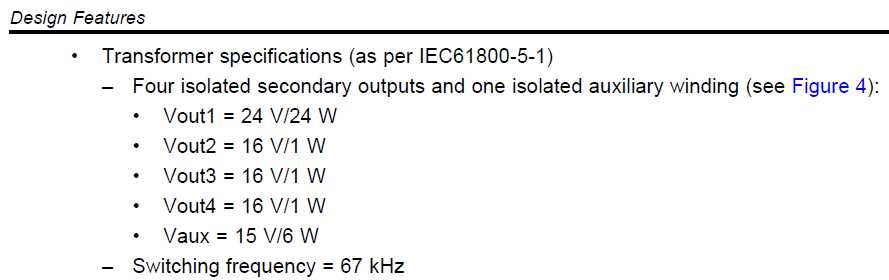If you have a related question, please click the "Ask a related question" button in the top right corner. The newly created question will be automatically linked to this question.

# UCC28711: UCC28711 Circuit design calculations

Part Number: UCC28711
Other Parts Discussed in Thread: TIDA-00173, , TIDA-00315, UCC28700

In my new design including the UCC28711 in a flyback power supply, I have some calculation questions:

I checked next documents:

a) TI reference design "400 to 690 VAC Input, 50-W Flyback Isolated power supply: TIDA-00173

b) TI reference design "100-V/200-VAC input 30W Flyback Isolated power supply: TIDA -00315

c) TI UCC28711EVM-160. Calculations checked according to document SLUA653B (UCC2870x)

My questions are related to calculations. I checked formulas in the three cases, and found some doubts/error/mistakes:

1) Power spec to Vauxiliary:

In case a) power is 15w, and in case b) is 6w.  In case c) is estimated 50mW.  Why is required so much power for Vaux, in case a and b?. What load is it calculated for?

2) DMAX duty cycle calculation:

In case a) used formula is : Dmax=(12*DMag(Vout+Vdg) )/ (Vin min-Vaon-VRCS)

In case b and c the formula is: Dmax= 1-DMAG- Fmax * Tr/2   . Which formula is correct? both?

3) NA/NS Turns ratio:

The formula is the same in the three cases, but Vddmin value is 16v in case a and 8v in case b and c.  Is a mistake case a)?? If this is so, next calculated values may be wrong

4) Iaux peak (auxiliary voltage peak current)

In case a and b, the used formula is the same: Iauxpk = (Pout * 2) / (Vout*Dmag), but in case c the formula is Iauxpk = ((Pout * 2) / ((Vout + Vde)*a2*Dmag).

I think the a2 parameter (Na/Ns) ratio in case c, is a mistake. Please confirm it. If this is so, next calculated values may be wrong

Thank you for you prompt answer. I look forward it to continue my design

• Hi Mike,

Regarding to Vaux power calculations, please help me indicate datasheet, app notes or any document using this formula: PIC = (2*Qg*fswmax) + VDD * Irun: I dont find it anywhere

I calculated the Vaux power for "400- to 690-V AC Input 50-W Flyback Isolated Power Supply", TIDA-00173, using this formula. The mosfet used in this case is STF2N95K5 (see attached Gate Source volt Vs Gate charge chart)According to this chart, using the same formula, gate charge is similar to the Vaux power calculated by you (approx 10nC for Vgs=10v). In that circuit the vaux power was 55mW, but in the TIDA-00173 vaux power is 15W. I suppose there is another reason for so much difference in Vaux power calculation, apart from mosfet selection. Please help me understand it.

All this comes from a new power supply I intend to design, and I have to define a Vaux power value

I suppose there is a mistake in your formula, and the correct answer is Dmax = 1 - Dmag - Fmax*Tr/2

• Hello Jean,

Equations for the estimate for the gate loss should be 2*Qg*fmax*Vgate+(Vdd*I run) . I had made a typo in the calculation. However, I am not sure how you are calculating 15W. The units would not have worked the mistake in the equation. With the correction to the formula, I estimate the power dissipation to be 74 mW. = (2*10nC*100kHz*16V) +2.6mA*16V =0.074W. It might be less than this depending on where the Aux voltage runs. Generally PWM ICs needs to dissipate less than 0.5W.

There was mistake in the formula for Dmax in the application note. Leaving out the fmax*Tr/2 would cause an error in the maximum duty cycle calculation and the design would run at a slower switching frequency with less duty cycle.

Regards,
• Hello again.

Please check the Ti documents of power supplies I am refering to (I attach the specs here): As you can see here, they have Vaux power value specs much higher than calculated with equations. I wanted to know the reason of it in order to take into account or not for my new design

TIDA-00173TIDA-00315I wanted to know which is the correct equation. The one you included as a response I think was not correct (please review it)

So to be sure, the formula is Dmax = 1 - Dmag - fmax * Tr/2. Please confirm it

About the turns ratio of aux winding to secondary the response was:  Np/Na = (Vdd(off) + Vd) / (Vout_min + Vd).

I think it is not correct, the first part of the equation should be Na/Ns. Please confirm it

Best regards

• Hello Jean,

The equation from aux ratio should be Na/Ns and in the text was described as aux winging to secondary winding. So you are correct that it should have been Na/Ns = (Vdd(off) + Vd) / (Vout_min + Vd).

Second the equation for Dmax = 1-Dmax-fmax*Tr/2 is correct and verified in the UCC28711 data sheet. www.ti.com/.../ucc28711.pdf Section 9.2.2.4 of the data sheet gives guidance on selecting a transformer using this method. The application note for the UCC28700 application note uses a similar calculation for Np/Ns = a1, www.ti.com/.../slua653c.pdf , this transformer selection guide is based on voltage second balance and Dmax calculation.

Every design is not the same and the power supply designer may use different approximations and design theory. Every design can be slightly different based on the design engineer’s experience and design approach and tradeoff they make. This might be why the tida designs are not giving the same value as the volt second balance approach.

Regards,

Mike
• Hello Jean,

I discussed this one of my colleagues today and it was mentioned to me that the tida designs in question had stated the aux power was designed for 15 W and 6 W. The power dissipation as you calculated was much less than the 50 mW that was estimated.

The tida designs are generally designed for a customer’s end application and most likely required the auxiliary power to be able to power more than the UCC28711 dissipated. This is not uncommon and is most likely why they designed there auxiliary power for more than 50 mW.

Regards,

Mike